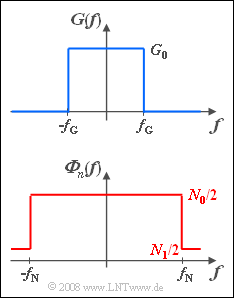# Exercise 5.8Z: Matched Filter for Rectangular PSD

(diff) ← Older revision | Latest revision (diff) | Newer revision → (diff)Spectrum  $G(f)\ \bullet\!\!\!-\!\!\!-\!\!\!-\!\!\circ\, \ g(t)$;
power-sprectral density ${\it \Phi}_n (f)$

The interference power-spectral density effective on a system can be assumed to be constant in range:

$$\it{\Phi} _n \left( f \right) = \left\{ \begin{array}{l} N_0 /2 \\ N_1 /2 \\ \end{array} \right.\quad \begin{array}{*{20}c} \rm{for} \\ \rm{for} \\\end{array}\quad \begin{array}{*{20}c} {\left| f \right| \le f_{\rm N} ,} \\ {\left| f \right| > f_{\rm N} .} \\\end{array}$$
• Here,  let the power-spectral density  $N_1$  in the outer region  $|f| > f_{\rm N}$  always be much smaller than  $N_0$.
• For example,  use the following values:
$$N_0 = 2 \cdot 10^{ - 6} \;{\rm{V}}^{\rm{2}} /{\rm{Hz}},\quad N_1 = 2 \cdot 10^{ - 8} \;{\rm{V}}^{\rm{2}}/ {\rm{Hz}}.$$

Such an interference signal  $n(t)$  occurs,  for example,  when the dominant interference source contains only components below the frequency limit  $f_{\rm N}$.    Due to the unavoidable thermal noise,  also for  $|f| > f_{\rm N}$  interference power-spectral density is  ${\it \Phi}_n(f) \ne 0$.

Further,  it holds:

• Let the spectrum  $G(f)$  of the useful signal  $g(t)$  also be rectangular according to the above diagram.
• Therefore,  $g(t)$  has the following curve with  $\Delta f = 2 \cdot f_{\rm G}$:
$$g(t) = G_0 \cdot \Delta f \cdot {\mathop{\rm sinc}\nolimits} \left( { \Delta f \cdot t} \right).$$
• Let the frequency response  $H_{\rm E}(f)$  of the receiver filter  (German:  "Empfangsfilter"   ⇒   subscript "E")  be optimally matched to the spectrum  $G(f)$  and the interference power-spectral density  ${\it \Phi}_n(f)$.
• That is,  let  $H_{\rm E}(f) = H_{\rm MF}(f)$  ⇒   "Matched Filter".
• Let the detection time be simplified  $T_{\rm D} = 0$  (acausal system description).

Notes:

• For numerical calculations always use the numerical values
$$G_0 = 10^{ - 4} \;{\rm{V/Hz}}{\rm{, }}\quad \Delta f = 10\;{\rm{kHz}}.$$

### Questions

1

Which of the following statements are valid under the condition  $f_{\rm N} > f_{\rm G}$?

 Applicable is the "matched filter for white noise". The matched filter output pulse is triangular. The matched filter output pulse is  $\rm sinc$–shaped. The matched filter output pulse is  $\rm sinc^2$–shaped.

2

What is the S/N ratio (SNR) of the detection signal  $d(t)$  for  $f_{\rm N} > f_{\rm G}$?

 $10 \cdot \lg \; \rho_d \ = \$ $\ \rm dB$

3

What SNR results for  $f_{\rm N} = f_{\rm G}/2$?  Interpretation.

 $10 \cdot \lg \; \rho_d \ = \$ $\ \rm dB$

### Solution

#### Solution

(1)  Solutions 1 and 3  are correct:

• For all frequencies  $|f| > f_{\rm G}$  at which the useful signal  $d_{\rm S}(t)$  has spectral components  $(G_d(f) \ne 0)$,
the interference power-spectral density is  ${\it}\Phi_n(f) = N_0/2$.
• Thus,  the frequency response of the matched filter is,  assuming  $T_{\rm D} = 0$:
$$H_{\rm MF} (f) = K_{\rm MF} \cdot G(f).$$
• In this case,  the optimal frequency response  $H_{\rm MF}(f)$,  just like  $G(f)$,  is rectangular with width  $\Delta f$.
• Thus,  for the useful component of the matched filter output signal  $d(t)$  holds:
$$d_{\rm S}(t)\quad \circ\!\!-\!\!\!-\!\!\!-\!\!\bullet\, \quad G(f) \cdot H_{\rm MF} (f).$$
• The product of two rectangular functions of equal width again yields a rectangular function.
• It further follows that the output pulse of the matched filter is also  $\rm sinc$–shaped.

(2)  With white noise one obtains:

$$\rho _d = \frac{1}{N_0 /2}\int_{ - \infty }^{ + \infty } {\left| {G(f)} \right|^2 \, {\rm{d}}f.}$$
• The integral yields the value  $G_0^2 \cdot \Delta f$.  It follows that:
$$\rho _d = \frac{G_0 ^2 \cdot \Delta f }{N_0 /2} = \frac{ 10^{ - 8}\,(\rm V/Hz)^2 \;\cdot10^4 \;{\rm{Hz}} }{10^{ - 6}\,\rm V^2/Hz} = 10^2 \quad \Rightarrow \quad 10\lg \rho _d \hspace{0.15cm}\underline { = 20\;{\rm{dB}}}.$$

(3)  In general,  the SNR for colored interference is:

$$\rho _d = 2 \cdot \int_0^\infty \frac{\left| {G(f)} \right|^2 }{{\it \Phi}_n (f)} \, {\rm{d}}f.$$
• As can be seen from the accompanying qualitative diagram,  that the integrand is piecewise constant for the given frequency responses.
• Thus,  with  $f_{\rm G} = 5 \; \rm kHz$  and  $f_{\rm N} = f_{\rm G}/2 = 2.5 \; \rm kHz$,  we obtain:
$$\rho _d = 2 \cdot 2.5\;{\rm{kHz}}\left( { \frac{10^{ - 2}}{\rm{Hz}} + \frac{1}{{{\rm{Hz}}}} } \right) = 5.05 \cdot 10^3 \quad \Rightarrow \quad 10\cdot\lg \rho _d \hspace{0.15cm}\underline {= 37.03\;{\rm{dB}}}.$$

Interpretation

• The matched filter frequency response  $H_{\rm MF}(f)$  has exactly the same shape as the integrand sketched above.
• If the constant  $K_{\rm MF}$  is chosen (arbitrarily) so that  $H_{\rm MF}(f) = 1$  in the range  $f_{\rm N} \le |f| \le f_{\rm G}$,  then for low frequencies  $(|f| < f_{\rm N})$:   $H_{\rm MF}(f) = 0.01$.  This means: The matched filter favors those frequencies that are only slightly affected by the interference  ${\it \Phi}_n(f)$.
• If instead we would use a filter  $H(f)$,  which gives equal weight to all frequencies up to and including  $f_{\rm G}$  (purple curve in the sketch below),
the following ratios would result:
$$d_{\rm S}( {T_{\rm D} } ) = G_0 \cdot 2 \cdot f_{\rm G} = 1\;{\rm{V}}, \quad \sigma _d ^2 = 10^{ - 6} \frac{{{\rm{V}}^{\rm{2}} }}{{{\rm{Hz}}}} \cdot f_{\rm G} + 10^{ - 8} \frac{{{\rm{V}}^{\rm{2}} }}{{{\rm{Hz}}}} \cdot ( {f_{\rm G} - f_{\rm N} } ) = 2.5 \cdot 1.01 \cdot 10^{ - 3} \;{\rm{V}}^{\rm{2}}$$
$$\Rightarrow \hspace{0.3cm} \rho _d = \frac {d_{\rm S}( {T_{\rm D} } )^2}{\sigma _d ^2} = \frac{1 \;{\rm{V}}^{\rm{2}}}{2.525 \cdot 10^{ - 3} \;{\rm{V}}^{\rm{2}}} = 396 \hspace{0.3cm} \Rightarrow \hspace{0.3cm}10 \cdot {\rm lg} \, \rho _d = 25.98 \, {\rm dB}.$$
• The signal–to–noise ratio is thus about  $11\ \rm dB$  worse than when using the matched filter for colored interference.